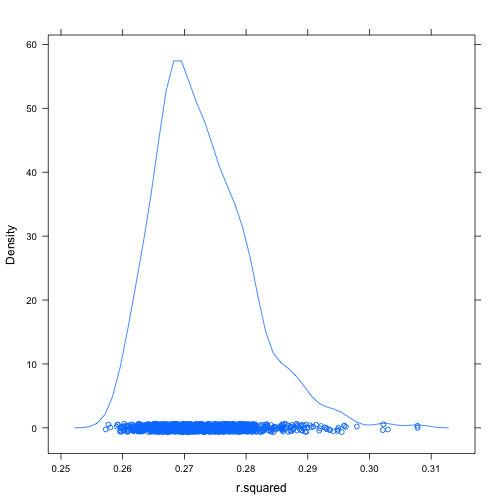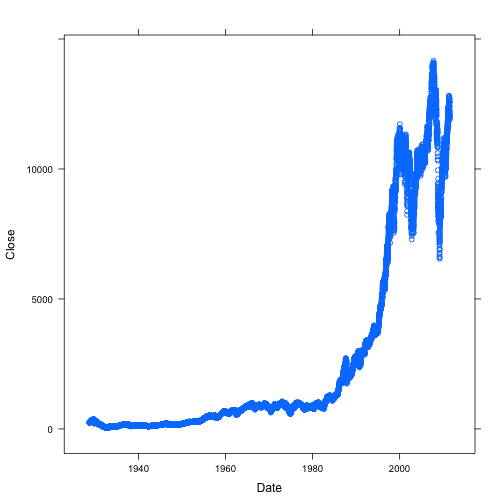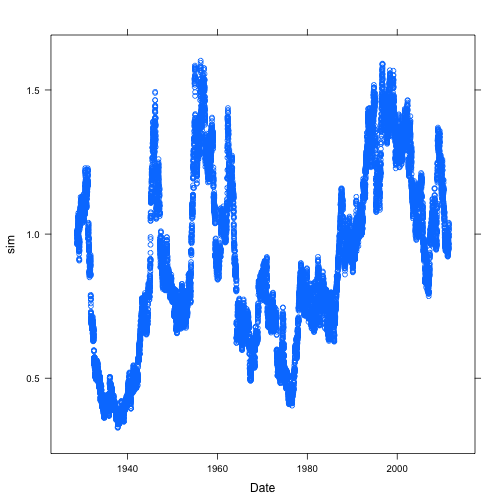# Friday March 29 Class Notes

### The Multi-World Metaphor

How to travel to the different worlds …

• Planet Sample: resampling
• Planet Null: shuffling
• Planet Alt: You need to construct such a world (we'll pick this up in a few days)

We've already done several examples using resampling — the idea is to see how much sampling variation there would be if the world were just like our sample.

Now to do shuffling.

Work with model coefficients and $$R^2$$ from a few models, with shuffling of an explanatory variable, or shuffling of the response.

Example: Sector of the economy and wages

mod = lm(wage ~ sector * sex + educ + exper, data = CPS85)
observedr2 = r.squared(mod)
observedr2

##  0.3113


Now go to Planet Null

s = do(1000) * lm(wage ~ shuffle(sector) * sex + educ + exper, data = CPS85)
densityplot(~r.squared, data = s)tally(~r.squared >= observedr2, data = s)

##
##  TRUE FALSE Total
##     0  1000  1000


What's the p-value?

Do the same on a coefficient and look at the two-tailed test.

### Stocks on Planet Null

fetchData("getDJIAdata.R")

## Retrieving from http://www.mosaic-web.org/go/datasets/getDJIAdata.R

##  TRUE

djia = getDJIAdata()  # djia-2011.csv is the basic file

## Retrieving from http://www.mosaic-web.org/go/datasets/djia-2011.csv

xyplot(Close ~ Date, data = djia)Look at the day-to-day differences in log prices:

dd = with(djia, diff(log(Close)))
mean(dd)

##  0.000191


Subtract out the mean, shuffle, cumulative sum, and exponentiate to create a realization:

ddnull = dd - mean(dd)
sim = exp(cumsum(shuffle(ddnull)))
xyplot(sim ~ Date, data = djia)### Drunk Driving Example

Planet Null:

• Set up a roadblock, pull out the people who are not drunk. Measure their results.

Planet Alt

• Take the drunk people, measure their results.

What's the decision threshold?

What's the

### App for playing with Significance and Power

fetchData("mHypTest.R")
mHypTest()  # by default, a coefficient